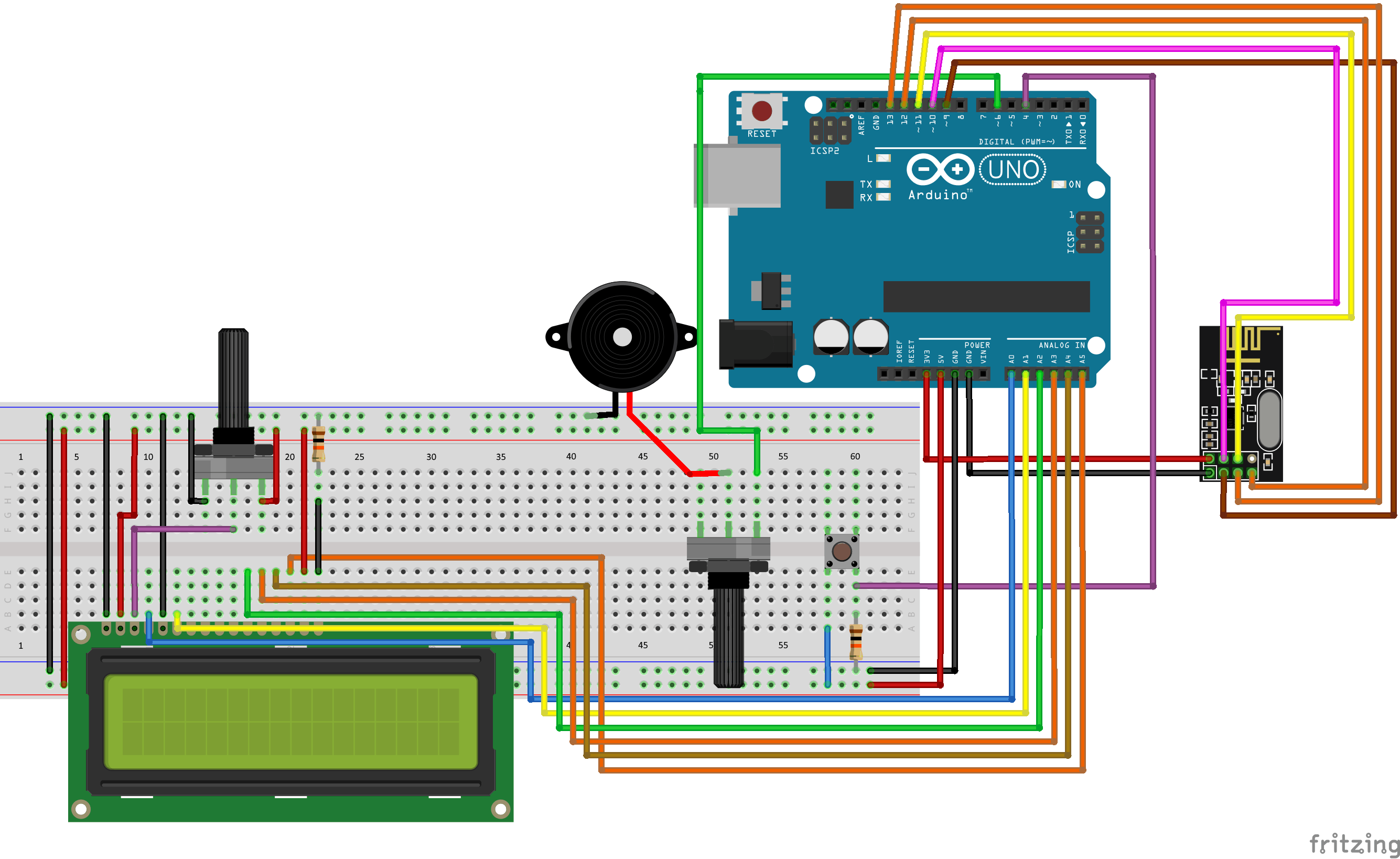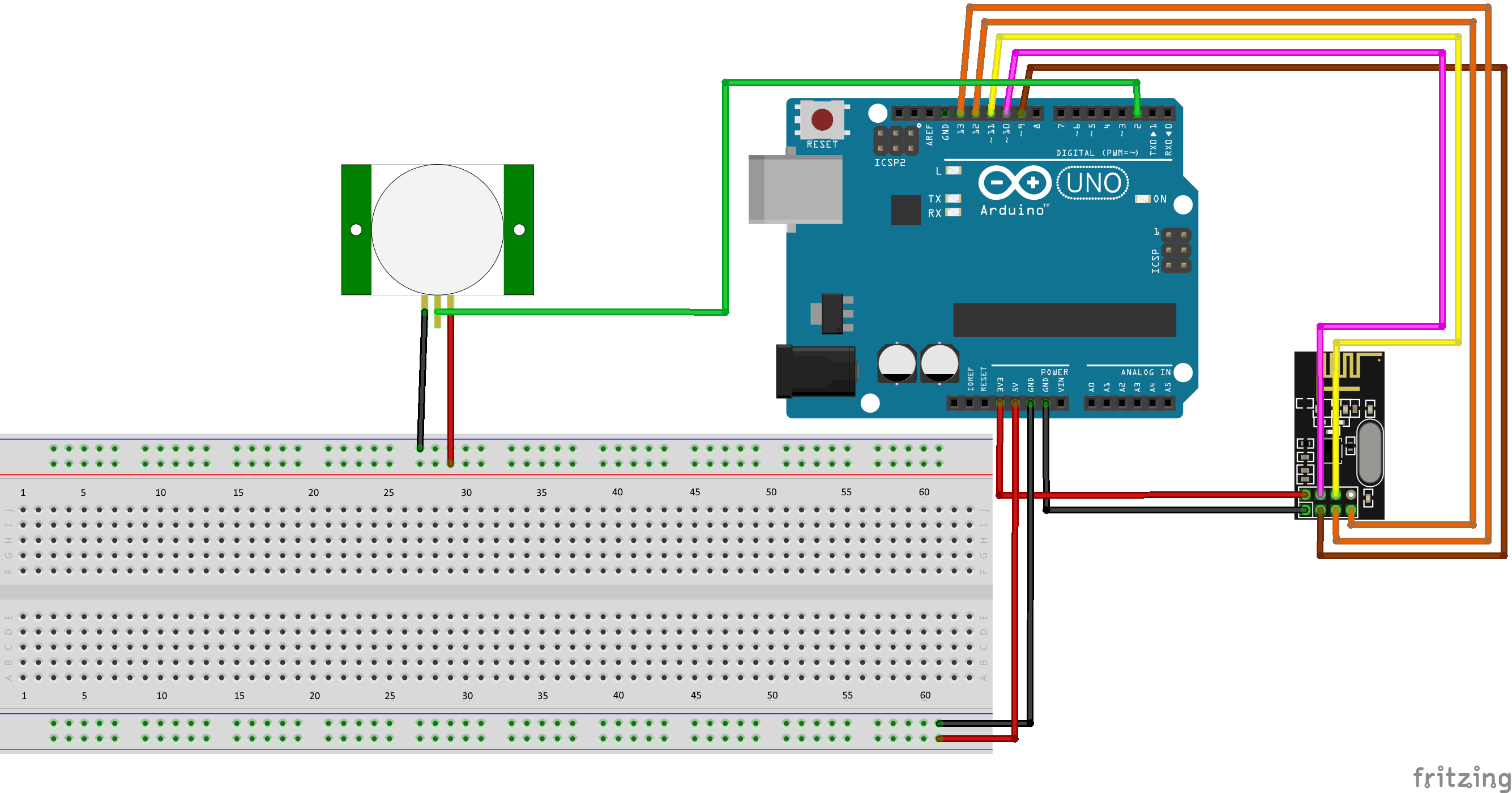# EXERCISE SOLUTIONS:

Challenge

This challenge requires us to modify both the PIR transmitter and the Control Center reciever code. First, we will change the lines in both the transmitter and receiver programs from bool message to int message. In the transmitter code, change the "1" in radio.write(message,1); to any integer you would like. In the example we will use the number "3587". Now, in the receiver code, update the if(message && armed) to if(message == 3587 && armed) (Replace "3587" with whatever integer you chose). Download the example code from "I Give Up" above to see the example of how this can be done!

Question 1

To display the packetLoss variable on the LCD, we have to add some code to the updateLCD function. At the end of the "if(millis()%500 < 50" if statement, we have to set the LCD cursor to the fourth line and print the packetLoss variable. Because the packetLoss variable is a decimal between 0 and 1, we should multiply the value by 100 in order to show it as a percentage. Download the example code from "I Give Up" above to see an example of how this can be done!

Question 2

To display the number of times the PIR sensor has been triggered, we can update the LCD similarly to how we did in Exercise 1. First, however, we need to create a variable which counts the number of times the PIR sensor has sent an "alarm" message to the control center. Now, in the updateLCD function, we set the LCD cursor to the third line and print the value of the new variable. Download the example code from "I Give Up" above to see an example of how this can be done!

Question 3

We can create a variable called lastRecievedTime. Every time a message is received, we will update this variable with the current time from millis. Now, in the loop we will check if the difference between the current time and the lastReceivedTime exceeds 5000 milliseconds. If so, we will activate the buzzer. Download the example code from "I Give Up" above to see an example of how to solve this exercise!